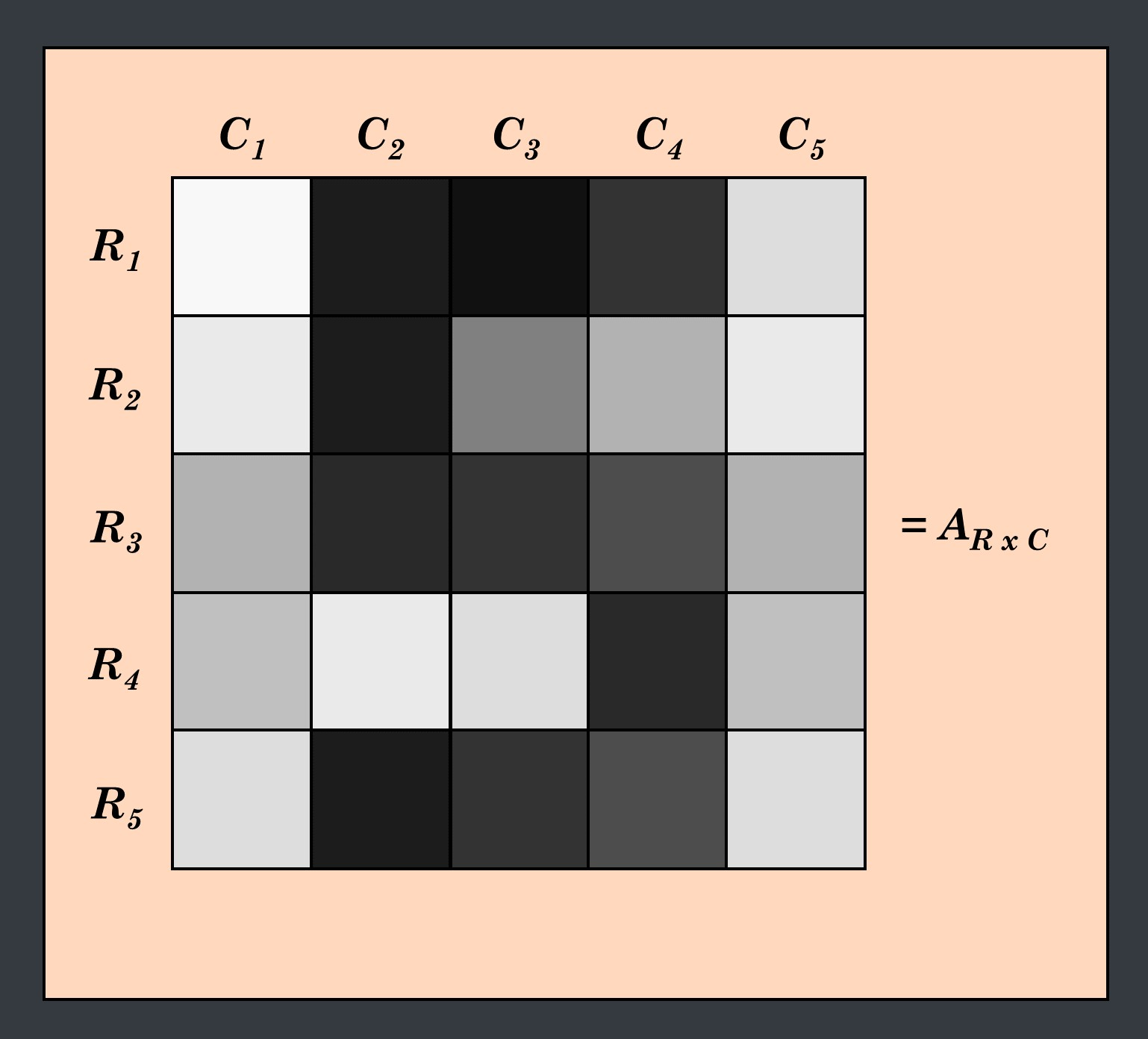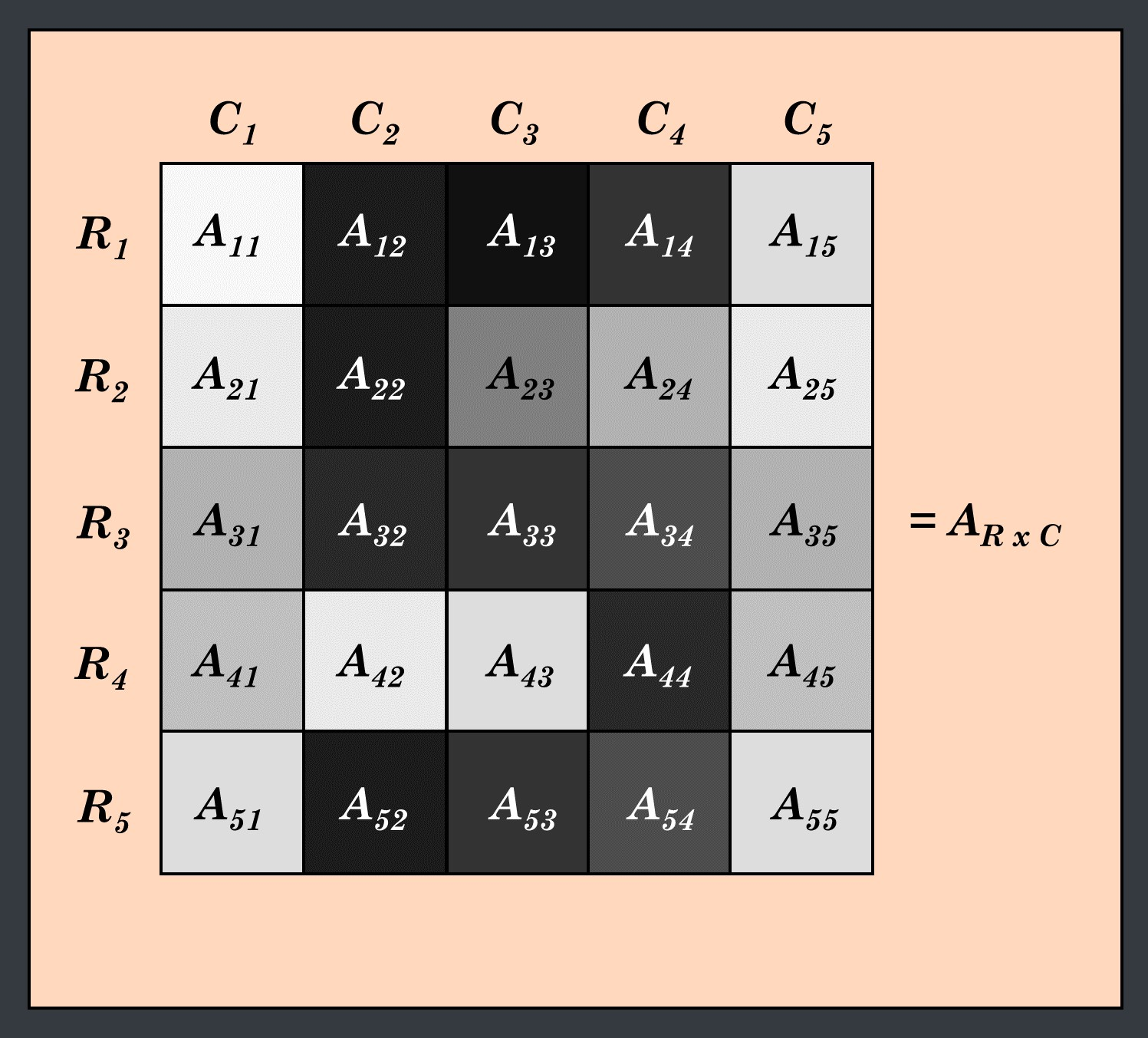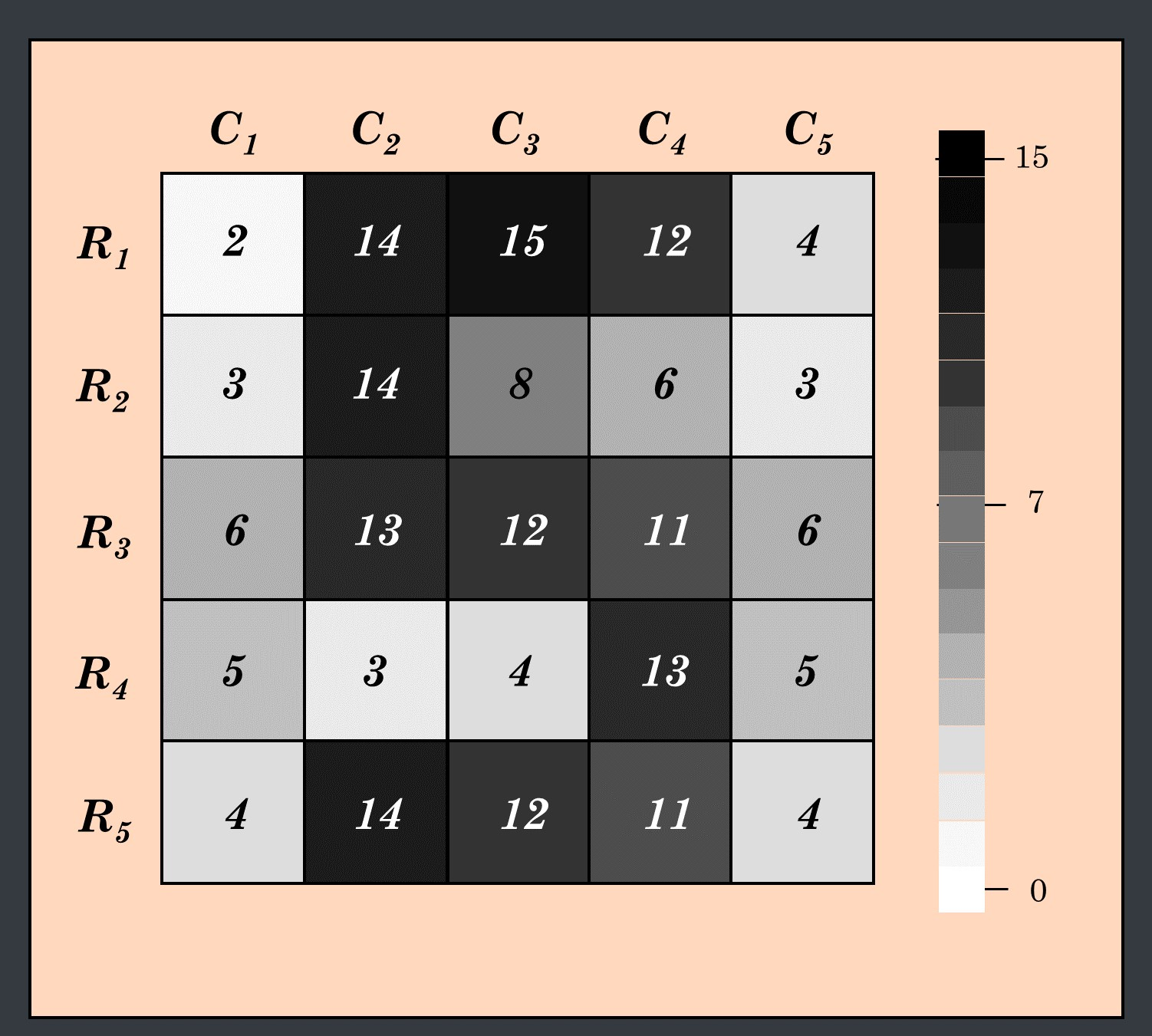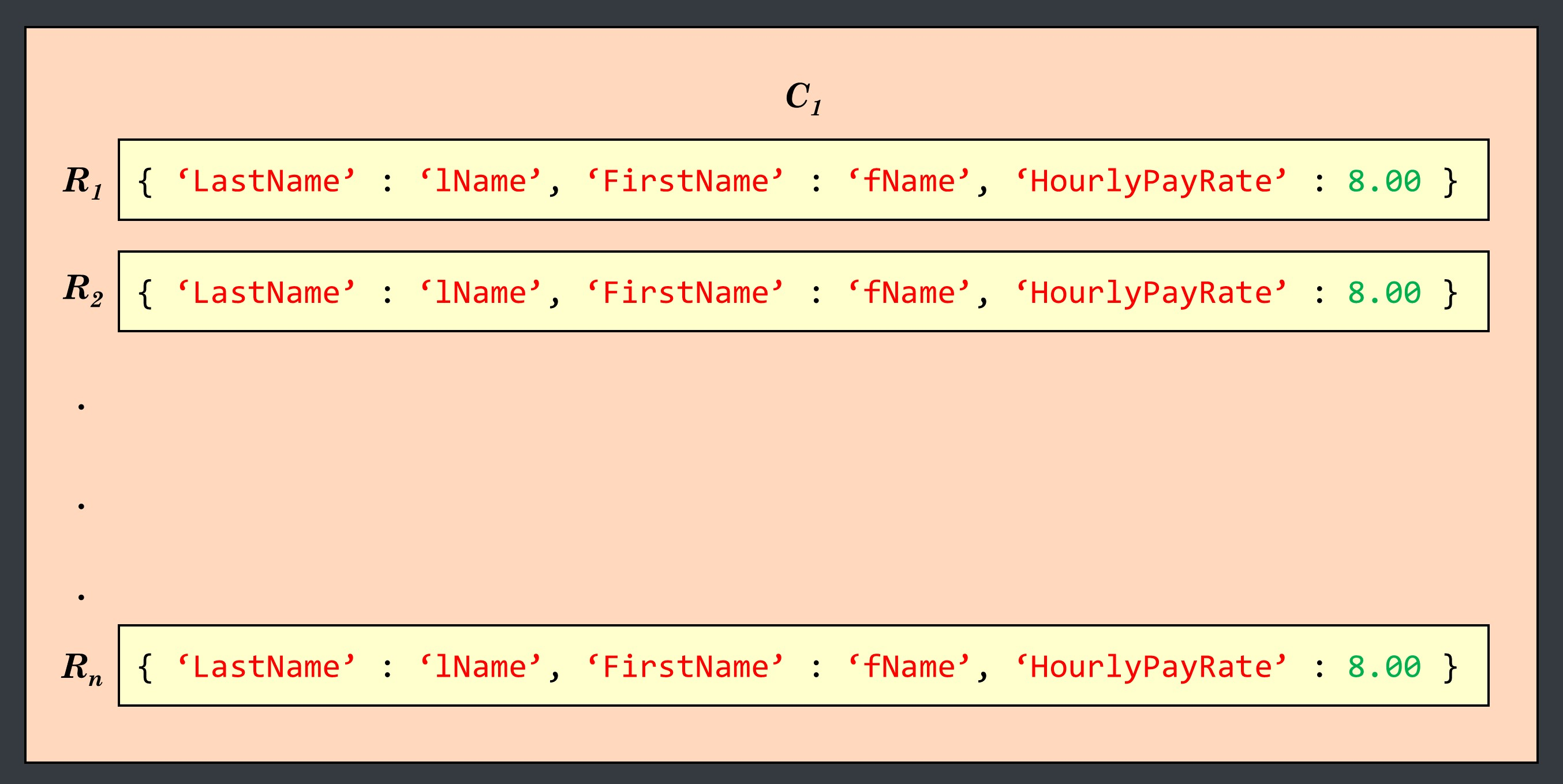#### The Array Data Structure

Arguably the most important data structure in programming is the array. Otherwise known as a matrix, an array is a way to order variables as elements in a specific, indexable, location.

Arrays allow us to store multi-dimensional data, like an entire excel spreadsheet, as a single variable. Almost any graph, chart, image, or table you see can be stored as an array, allowing for easily manipulation, fitting, and visualization of data. Because of their similarity to mathematical matrices, it is simple to apply algorithms to arrays by treating them as matrices, which are the fundamental building blocks to mathematics fields like statistics and probability. These two fields are the basis of scientific data analysis, and thus using arrays for scientific programming is a natural match. Particularly in python, there is an extremely powerful package called NumPy, i.e. Numerical Python, which utilizes the power of arrays to do fast scientific data analysis. Further, NumPy has lots of tools to make building and working with arrays simple, and if you are using python for scientific programming/data analysis, you should
become very familiar with NumPy.

As already mentioned, it is nice to think of arrays as matrices, and then apply our knowledge of matrix/linear algebra to understand how to work with arrays. However, if your matrix knowledge is limited, it may be difficult to jump into utilizing arrays (and thus programming) to its fullest. This blog post is to assist newcomers to python in understanding how to work with arrays, and to see the benefit of storing data as an array data structure, rather than individual variables.

To go along with this blog post, I have created a Jupyter Notebook which can be found in my blog repo here. This notebook illustrates the ideas here in python code, as well as contains additional information/examples about arrays, and can serve as a standalone reference.

##### (I) Images as ArraysLets look at the gray-scale letter "S" above as an example to see how the information stored in an image can be readily stored in an array. This image is exaggerated to be broken into large pixels, where in real images, the pixels are much smaller.

We can place a grid over the image such that within each square of the grid, there is a single pixel of a single shade of gray.A grid is a great depiction of a matrix, as it has numbered rows (R1-R5), and columns (C1-C5). We can call this matrix AR x C, where R is the number of rows of the matrix, in this case 5, and C is the number of columns of the matrix, 5, and we call A a "five-by-five" matrix.The rows and columns of a matrix serve as a type of longitude and latitude to locate a specific item at a specific location. We call these locations elements, and the longitude and latitude are the row index and the column index. Further, we use a specific notation to indicate the location of a specific element in a matrix. Using our A matrix, the notation for the element in the top left corner would be A11 because it is in R1 (row 1) and C1 (column 1). In general, we say that an element is defined as Aij where i is the row number, and j is the column number, and we say "element Aij is in the ith row and the jth column.

Using this notation, we can assign an element to each pixel.Now we know how an image can be broken up into pixels, and how these pixels correspond to an element in a matrix, however how do we store these elements in an array?

Each pixel is a specific shade of gray, and thus we can assign it an "intensity", or in other words a value which tells us how black the pixel is. I picked 15 shades of gray and numbered them from 0 (being white) to 15 (being black), and then assigned a number 1-15 to each colored pixel. This value is what is stored in each element of an array of this image.We can create and view an image like this as an array in python fairly simply as illustrated in the notebook. Briefly, we first need to create an empty array, A by using the NumPy function zeros() which creates an array of the desired size with each element as a zero. This is a valuable way to create arrays, because it is more efficient for the computer to edit an already available array in memory than it is to append new values to a list. This may not seem apparent now, but when working with large arrays and time-consuming algorithms, this method of array creation saves you in computational cost.

After we create the array there are several ways to replace/edit the zeros with the desired values which I elaborate on in the notebook in section (III) Array operations. For this example, I have chosen to create five lists for the five rows of the A matrix, each containing the five values in the different columns. Using these lists, I then create a for-loop which replaces the zeros for each row with the elements of the five lists that I generated. This creates the 5x5 array which represents the A matrix discussed above. we can then visualize this with matplotlib, and see that it is an identical replication of the figures shown previously.Once we have these pixel values in an array, we can perform operations on them to change the intensity of each pixel, or delete pixels.

##### (II) Array of Dictionaries

Another powerful aspect of arrays is that they can store integer, float, string, and dictionary variable types, making it easy to store both numerical data and descriptive text in a dataset. To illustrate how powerful this is, we will explore an array of dictionaries.

For this example, we will be creating an array which stores a dictionary as each element. This type of array is useful for when you have several pieces of similar information (i.e. first name, last name, address, etc.) for different users, employees, patients, etc. Dictionaries allow us to use what is called a key to refer to specific values. This is useful because you can call the key for several different dictionaries and edit the corresponding values at once. This is extremely powerful for adding or calling information about users, and especially for changing something like base pay rate across the board.

We can create an array like this with a for-loop using NumPy's array() function.

``numberEmployees = 3employeeArray = np.array([{'LastName':'lName',                           'FirstName':'fName',                           'HourlyPayRate':8.00} for i in range(numberEmployees)])``

Which creates an array which only has one column, and has as many rows as we designated by the numberEmployees variable.We can extract out the information for a specific key using a for-loop. For example:

``for i in range(employeeArray.shape):    print employeeArray[i]['LastName']``

Will print the value at the 'LastName' key for each element in the array.

Hopefully this post and reference notebook have shed some light on the value and importance of using array data structures, and some insight into how to create and manipulate them. I will continually advocate for python and it's various scientific packages, including NumPy, as they not only make working with data sets large and small easier and more efficient, but allow you to manipulate and process data in ways that couldn't be possible without the use of a programming language.

All thoughts and opinions are my own and do not reflect those of my institution.